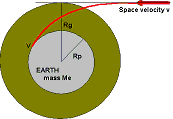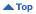Educational
Saturday, Dec 09 2023 11:28 UT

Space Debris

# Earth Cross Section for Meteoroids

For a projectile to hit a target it normally must impact within an area which is the cross-sectional area of the target. For a spherical body, this area isr2 where r is the radius of the sphere. However, in the case of a meteoroid or an asteroid impacting the Earth (or another planet), the appropriate cross-sectional radius is not the physical radius of the Earth, but its gravitational radius, which depends upon the impactor velocity.This is because the Earth's gravity will attract an impactor that might be approaching on a near miss orbit. Imagine we have an impactor that in the absence of gravity has a space velocity v, and is on a trajectory with a distance Rg from the Earth's centre. Because of gravity the trajectory will curve toward the Earth and impact with a velocity V.

This impact will be tangential if Rg is the gravitational radius of the Earth (for the space velocity v (ie the body just impacts the planet). We can use the laws of conservation of momentum aned conservation of energy to determine Rg.

v Rg = V Rp (conservation of momentum)
v2/2 = V2/2 - G Me / Rp (conservation of energy)

where G is the universal gravitational constant and Me is the mass of the Earth. Solving these two equations simultaneously we find that:

Rg = Rp √[ 2 G Me / ( v2 Rp ) + 1 ]

The table below presents values of Rg (as a function of the space velocity), both in kilometres and as a fraction of the Earth's radius. Also shown are the fractional area, and the collision velocity.

Space
vel(km/s)
Collision
vel(km/s)
Effective
Fractional
Fractional
area
211362375.6832.26
412189422.978.81
512156302.456
613134942.124.47
814109651.722.95
101595711.52.25
151979581.251.56
202373091.151.31
252769891.11.2
303268091.071.14
353766981.051.1
404266251.041.08
454665741.031.06
505165381.021.05

Material prepared by John Kennewell